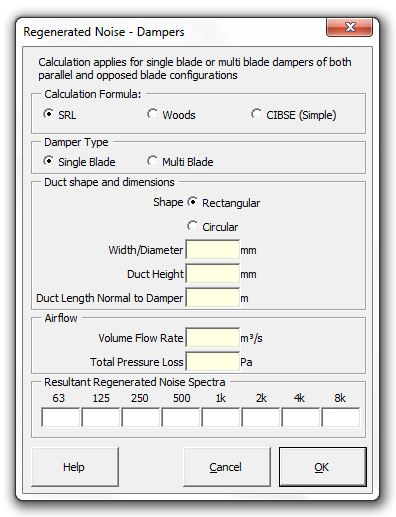### Strutt Help

Regenerated Noise - Dampers    1/1

Strutt|Mechanical Services|Regenerated Noise|Damper inserts sound power levels into the active row of the worksheet.Regenerated noise can be calculated using the Woods, SRL or CIBSE methods.

Woods
calculates regenerated noise from dampers using:

L_W = K + 10log_10 S + 55log_10 V - 45

Where:
L_W is the total sound power level generated by the actual fitting, dB
K is constant, datum power level of the fitting (given below), dB
S is the actual duct area, m2
V is the actual velocity, m/s

Values of K (from Table 5.2 Woods):

 Damper K, dB Open 44 15º closed 53 45º closed 65

Octave band sound power levels are calculated by applying the following corrections (Table 5.2, Woods):

 63 Hz 125 Hz 250 Hz 500 Hz 1 kHz 2 kHz 4 kHz 8 kHz -6 -6 -7 -7 -8 -10 -17 -29

SRL calculates regenerated noise from dampers using:

L_W(f_0) = K_D - 18 + 10log_10(f_0) + 50log_10(U_C) + 10log_10(S) +10log_10(D)

Where:
f_0 is the octave band centre frequency, Hz
U_C is the flow velocity in the constricted part of the field between the blades determined using:

U_C = Q/(S*BF), where BF is the Blockage Factor given by:

• For multi-blade dampers: BF = (C^(1//2)-1)/(C-1) if C != 1, or BF = 0.5 if C = 1
• For single-blade dampers: BF = (C^(1//2)-1)/(C-1) if C < 4, or BF = 0.68 C^(-0.15) - 0.22 if C > 4
where,

C = (1.67 Delta p)/(Q/S)^2

Q is the volume flow rate, m3/s
p is the pressure loss, Pa
S is the cross sectional area of the duct, m2
D is the duct height normal to the damper axis, m
n is the number of turning vanes
K_D is the characteristic spectrum which is given by:

K_D = 5.0 - 10.7log_10(St), for St < 25
K_D = 40.2 - 35.9log_10(St), for St > 25
St is the Strouhal number, given by:

St = (f_0 D_i)/U_i

where:
D_i is the characteristic duct dimension
U_i is the flow velocity of duct element i.

The simple CIBSE method calculates the regenerated noise using:

L_W=K_t+10log(S)+60log(V)+C+Delta L_("turb")

where:
K_t is a constant depending on the duct fitting type. K_t=-5 for a butterfly damper
S is the duct cross-sectional area (m˛)
V is the duct velocity (m/s)
C is a spectrum correction term (from Table 5.1 CIBSE Guide B)
DeltaL_(turb) is a user-inputted correction (dB) increasing the sound power levels to account for turbulent inflow onto the fittings.

Note there is a more-detailed CIBSE method based on the pressure loss factor etc, the Regenerated Noise from Duct Fittings (Detailed) calculation, which can be used if the pressure drop and loss factor of the fitting are known

References:

• Woods Practical Guide to Noise Control, p101.
• SRL Noise Control in Building Services, pp244-250
• CIBSE Guide B Section 5.5.1